Ex 4.4

Chapter 4 Class 12 Determinants
Serial order wise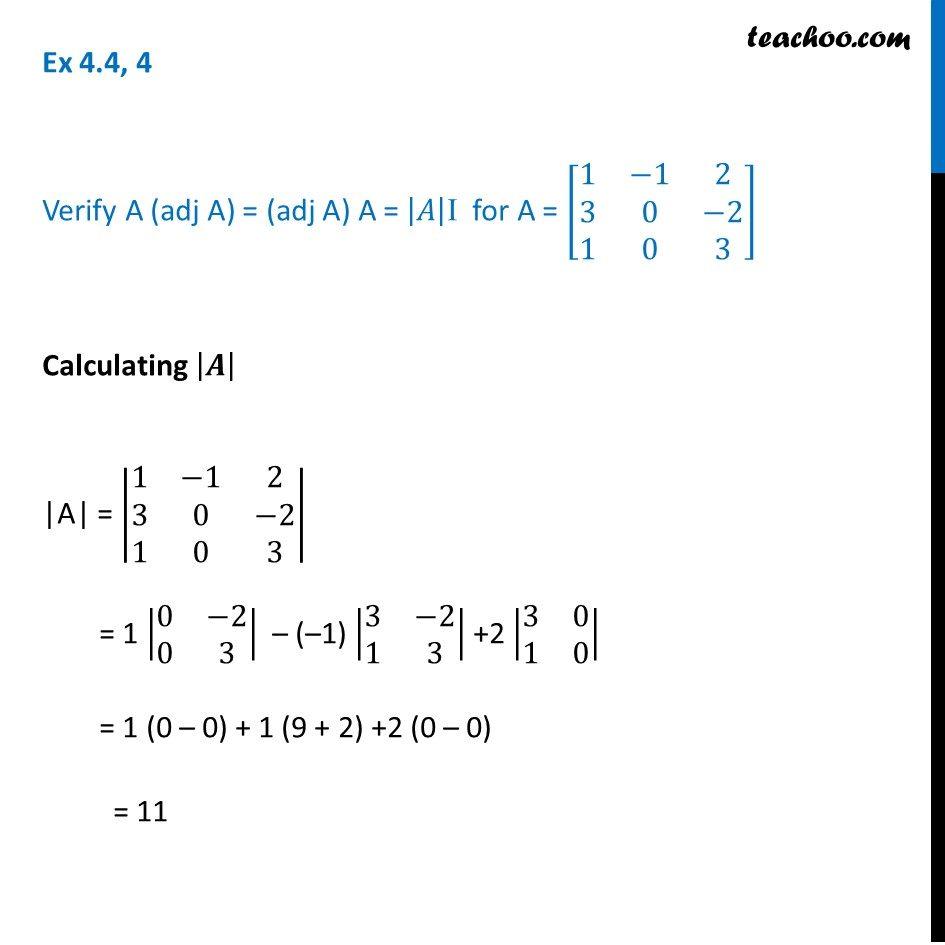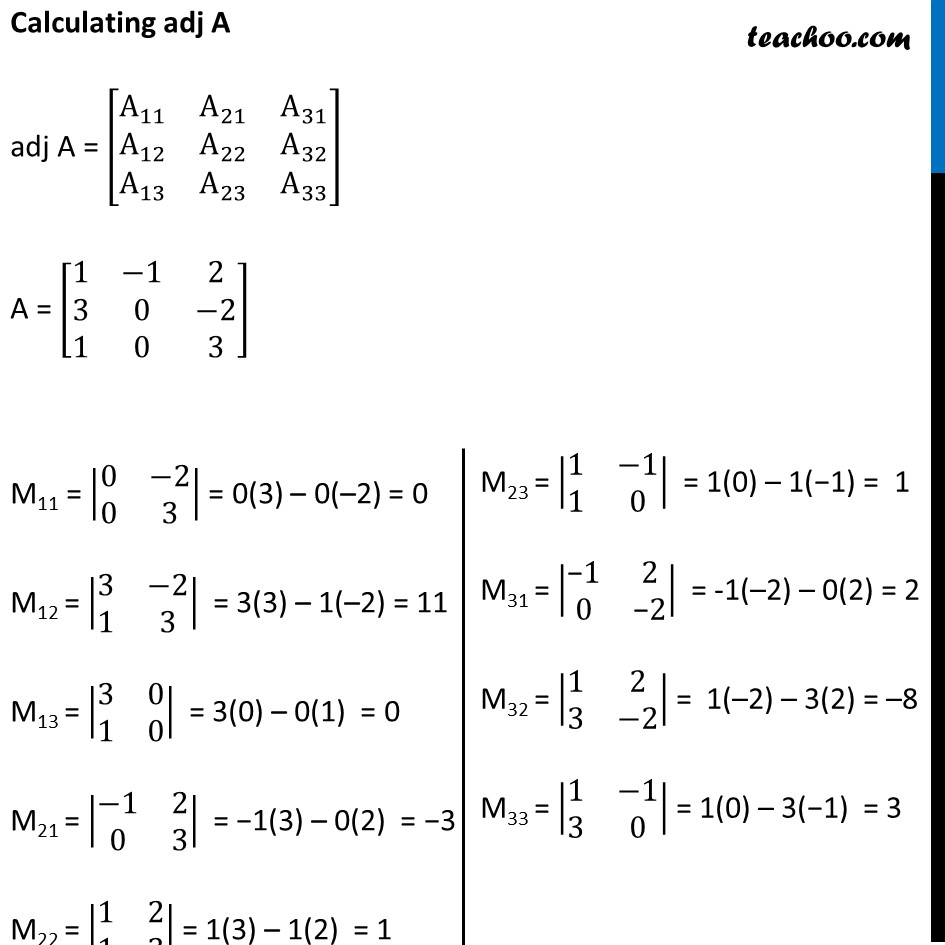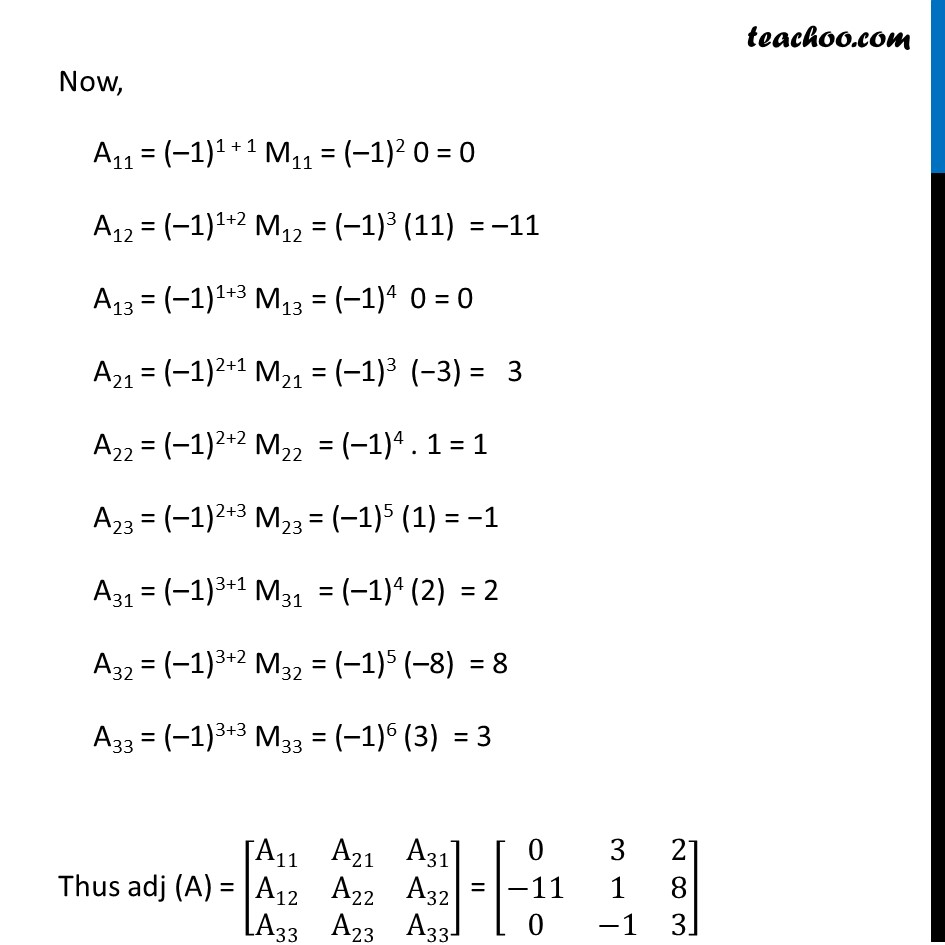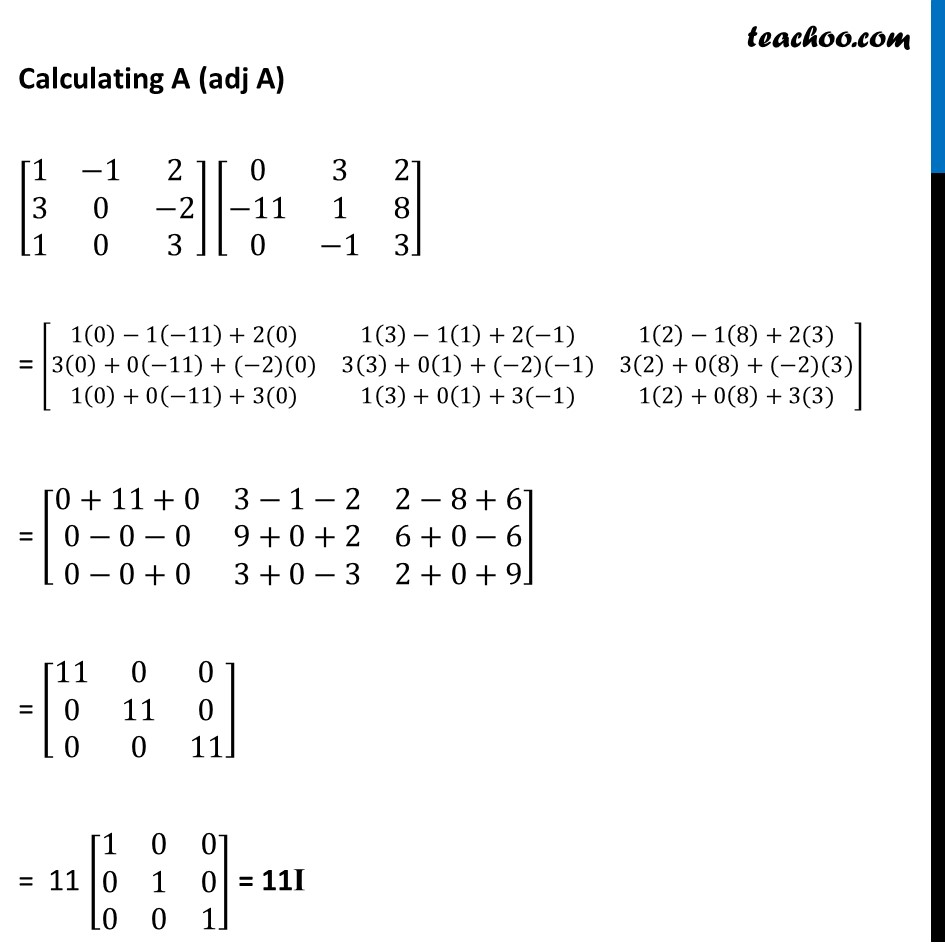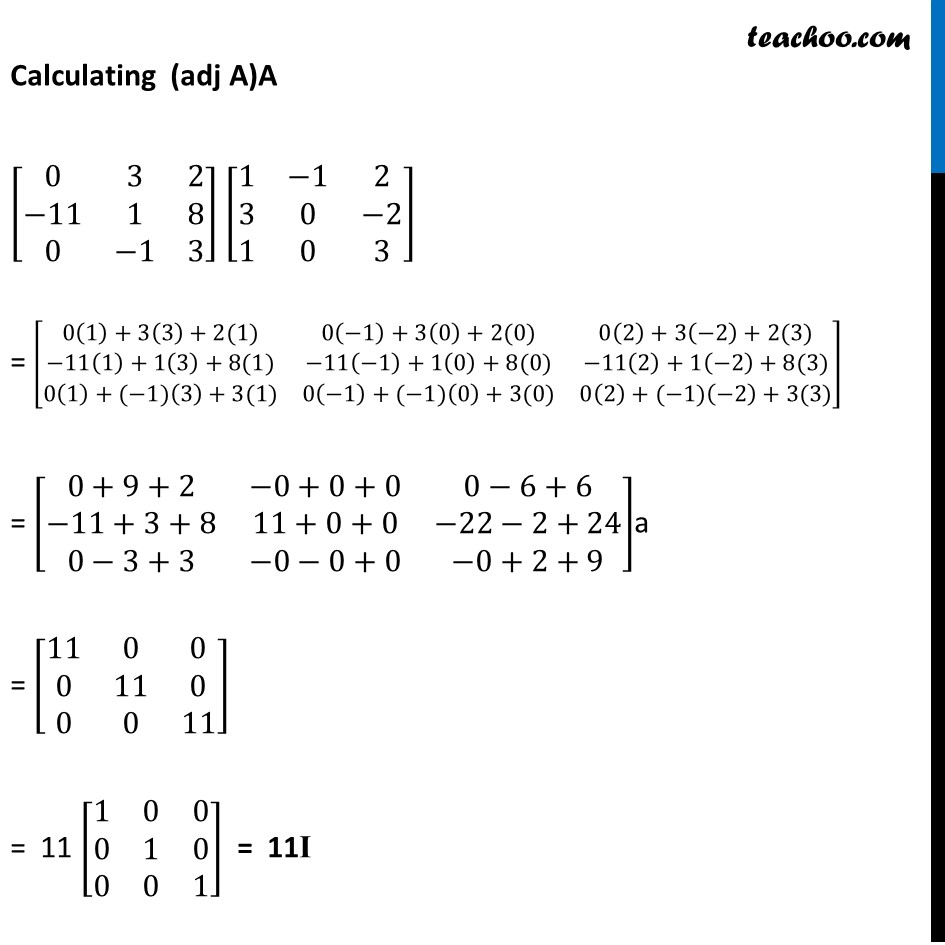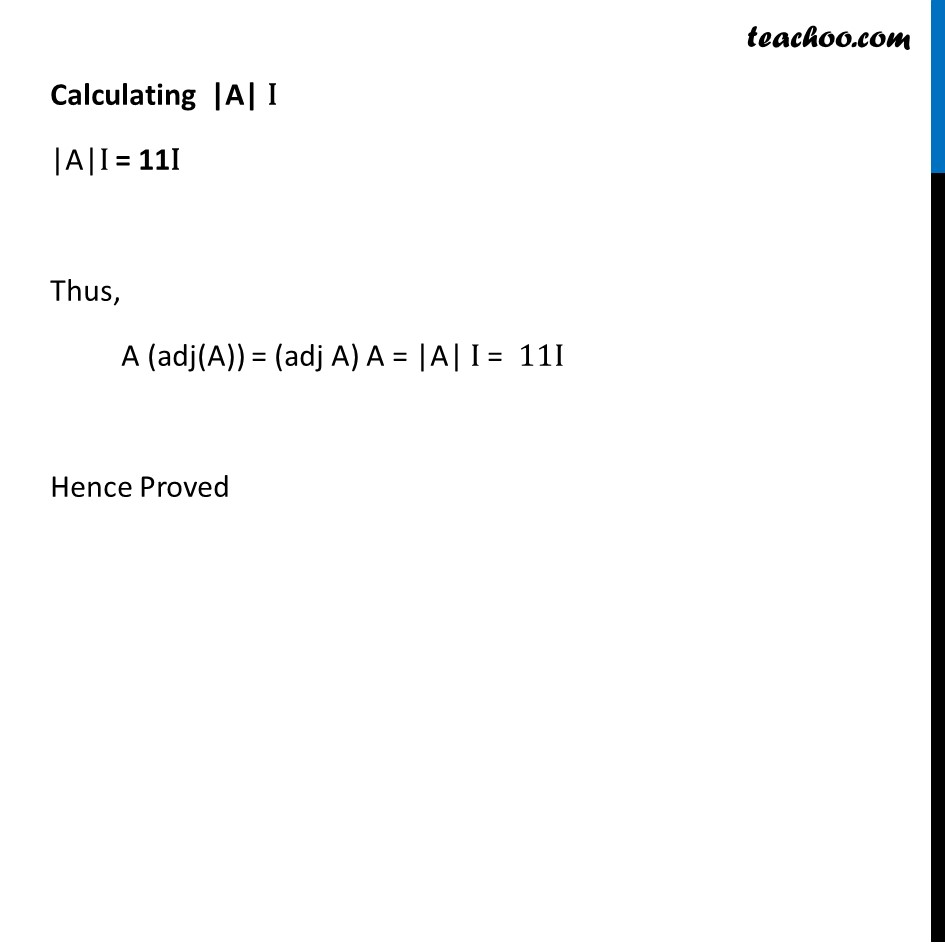Learn in your speed, with individual attention - Teachoo Maths 1-on-1 Class

### Transcript

Ex 4.4, 4 Verify A (adj A) = (adj A) A = |𝐴|I for A = [■8(1&−1&2@3&0&−2@1&0&3)] Calculating |𝑨| |A| = |■8(1&−1&2@3&0&−2@1&0&3)| = 1 |■8(0&−2@0&3)| – (–1) |■8(3&−2@1&3)| +2 |■8(3&0@1&0)| = 1 (0 – 0) + 1 (9 + 2) +2 (0 – 0) = 11 Calculating adj A adj A = [■8(A_11&A_21&A_31@A_12&A_22&A_32@A_13&A_23&A_33 )] A = [■8(1&−1&2@3&0&−2@1&0&3)] M11 = |■8(0&−2@0&3)| = 0(3) – 0(–2) = 0 M12 = |■8(3&−2@1&3)| = 3(3) – 1(–2) = 11 M13 = |■8(3&0@1&0)| = 3(0) – 0(1) = 0 M21 = |■8(−1&2@0&3)| = −1(3) – 0(2) = −3 M22 = |■8(1&2@1&3)| = 1(3) – 1(2) = 1 M23 = |■8(1&−1@1&0)| = 1(0) – 1(−1) = 1 M31 = |■8("−" 1&2@0&"−" 2)| = -1(–2) – 0(2) = 2 M32 = |■8(1&2@3&−2)| = 1(–2) – 3(2) = –8 M33 = |■8(1&−1@3&0)| = 1(0) – 3(−1) = 3 Now, A11 = (–1)1 + 1 M11 = (–1)2 0 = 0 A12 = (–1)1+2 M12 = (–1)3 (11) = –11 A13 = (–1)1+3 M13 = (–1)4 0 = 0 A21 = (–1)2+1 M21 = (–1)3 (−3) = 3 A22 = (–1)2+2 M22 = (–1)4 . 1 = 1 A23 = (–1)2+3 M23 = (–1)5 (1) = −1 A31 = (–1)3+1 M31 = (–1)4 (2) = 2 A32 = (–1)3+2 M32 = (–1)5 (–8) = 8 A33 = (–1)3+3 M33 = (–1)6 (3) = 3 Thus adj (A) = [■8(A11&A21&A31@A12&A22&A32@A33&A23&A33)] = [■8(0&3&2@−11&1&8@0&−1&3)] Calculating A (adj A) [■8(1&−1&2@3&0&−2@1&0&3)] [■8(0&3&2@−11&1&8@0&−1&3)] = [■8(1(0)−1(⤶7−11)+2(0)&1(3)−1(1)+2(−1)&1(2)−1(8)+2(3)@3(0)+0(⤶7−11)+(−2)(0)&3(3)+0(1)+(−2)(−1)&3(2)+0(8)+(−2)(3)@1(0)+0(⤶7−11)+3(0)&1(3)+0(1)+3(−1)&1(2)+0(8)+3(3))] = [■8(0+11+0&3−1−2&2−8+6@0−0−0&9+0+2&6+0−6@0−0+0&3+0−3&2+0+9)] = [■8(11&0&0@0&11&0@0&0&11)] = 11 [■8(1&0&0@0&1&0@0&0&1)] = 11I Calculating (adj A)A [■8(0&3&2@−11&1&8@0&−1&3)] [■8(1&−1&2@3&0&−2@1&0&3)] = [■8(0(1)+3(3)+2(1)&0(−1)+3(0)+2(0)&0(2)+3(−2)+2(3)@−11(1)+1(3)+8(1)&−11(−1)+1(0)+8(0)&−11(2)+1(−2)+8(3)@0(1)+(−1)(3)+3(1)&0(−1)+(−1)(0)+3(0)&0(2)+(−1)(−2)+3(3))] = [■8(0+9+2&−0+0+0&0−6+6@−11+3+8&11+0+0&−22−2+24@0−3+3&−0−0+0&−0+2+9)]a = [■8(11&0&0@0&11&0@0&0&11)] = 11 [■8(1&0&0@0&1&0@0&0&1)] = 11I Calculating |A| I |A|I = 11I Thus, A (adj(A)) = (adj A) A = |A| I = 11I Hence Proved